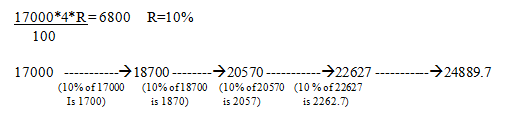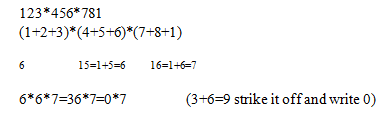Use coupon code TOP15 on all courses

# 5 Tricks for Quantitative Aptitude Section

First and foremost the most important trick is to solve using options

Ax²+Bx+C=0
Split the middle terms (B) and product terms (AC) mentally.
For example: 187 x²+6x-1=0 …………..quadratic equation
-187=17 x -11 after getting the prime factors 17 and 11 I realized 17-11=6
factors 17 and -11

reverse signs -17 and 11
divide by A: -17/187 and 11/187 so the factors are x = -1/11 and 1/17  153y²-26y+1=0
+153= 3 x 3 x 17 to get -26 the factors can be arranged as -9-17=-26 and -9 x -17=+153  factors -9 and -17
reverse signs 9 and 17
divide by A: 9/153 and 17/153 factors are: y =1/17 and 1/9
y>=x.

### 2. Profit , loss and discount

Profit and Loss questions can be simply treated as ration questions
If said 20 perc discount = 20/100
So bring it in SP: CP terms i.e. 80:100 SP is 80 CP is 100 SP-CP= 80-100=20 (discount)
Similarly marked up by 5perc is 5/100 but since it’s marked up it becomes 105:100 i.e. SP = 105
CP= 100 .SP-CP=105-100.
A shopkeeper marks up his goods by 20% and gives a discount of 5%.Also he uses a false balance which reads 1000gms for 750gms .What is his total profit percentage.
Mark up of 20% is 120/100
Discount by 5% is 95 /100
Claimed weight /actual weight 1000/750 (analyze if situation of profit num>den
If situation of loss num<den)Profit percent: 13/25 x 100=52%

### 3. Ratio and proportion

The trick is in taking it as a unit
A mixture contains wine and water in the ratio 3:2 and another contains them in the ratio 4:3.If they are mixed in the ratio 2:3, what is the ratio of wine and water in the resultant mixture?

wine water unit
1st mixture 3 x7 2 x7 5 x7
2nd mixture 4 x5 3 x5 7 x5

• Make the units equal.
• Multiply the final equal table with the ratio in which they are mixed (2:3).

wine Water unit
21 14 35
20 15 35

Wine Water Unit
42 28 70
60 45 105
102 73
Thus resultant ratio 102:73

### 4. Compound Interest

Rather than calculating compound interest using formula just use percentage method. The simple interest accrued on an amount of Rs 17000 at the end of four years is Rs 6800.What would be the compound interest accrued on the same amount at the same rate in the same periodInterest accrued: 1700+1870+2057+2262.7 = 7889.7

### 5. Simplification – Digit sum

Firstly add all the digits of a number to bring a single no.
Rule if sum is 9 strike it off and write 0
Any other no. is considered
Secondly try and find the units digit which will further make the answer a 100 perc sure.

### Example :

#### Ques 1.

….. 123 x 456 x 781
a)43804728
b) 53804728
c)53804528
d) 33804958

Ans.So digit sum is zero and unit’s digit is 8
Which ever option has digit sum as zero is the ans
4+3+8+0+4+7+2+8 = 36 (9=0)
5+3+8+0+4+7+2+8 = 37 (10=1)
5+3+8+0+4+5+2+8 = 35 (8)
3+3+8+0+4+9+5+8 = 40 (4)

#### Ques 2

6269+0.75 x 4444+0.8 x 185
a)9759
b)9750
c)9740
d)9755
e)9655

Ans:= 6269+3333+4 x 37
Digit sum : 5+3+4 x 1 = 5+3+4 = 3
Unit’s digit :0
9+7+5+9 = 3
9+7+5+0 = 3
9+7+4+0 = 2
9+7+5+5 = 0
9+6+5+5 = 7
Ans is clearly b where digit sum is 3 and units digit is 0.

#### Latest Courses on BankExamsToday